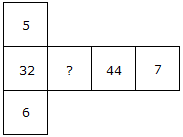# Verbal Reasoning - Character Puzzles - Discussion

### Discussion :: Character Puzzles - Character Puzzles 1 (Q.No.48)

48.

Which one will replace the question mark ?[A]. 33 [B]. 38 [C]. 32 [D]. 37

Explanation:

(5 x 6) + 2 = 32

(7 x 6) + 2 = 44

(7 x 5) + 2 = 37.

 Raja said: (Jun 13, 2016) Good. I like to solve this type of questions. Thank you.

 Bishen said: (Mar 15, 2019) I am not understand it. Please someone explain me in a simple way.

 Geeta Rajput said: (Sep 12, 2019) Would you please tell me in details.

 Arun Patil said: (Mar 20, 2020) Hi friends, it needs to calculate the end digit to end digit. First multiple last two end digits (5*6)+2=32, next multiple with one end digit to another so we need to consider right side given 7 and multiple with below end given value 6 ie. (7*6)+2=44, similarly consider another top side given value and multiple with 7 i.e. (7*5)+2=37.

 Rohini said: (Sep 29, 2020) Thanks @Arun patil.